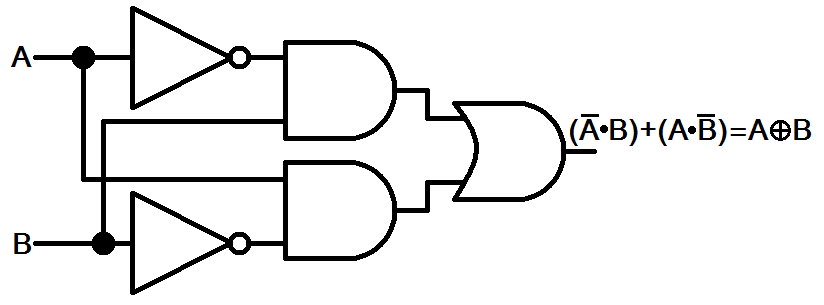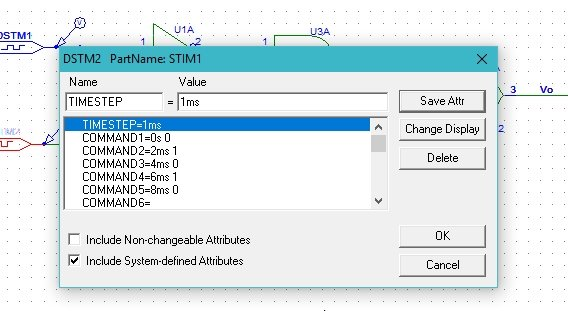# Digital circuits simulation using PSpice: tutorial 10

In this tutorial I will explain you the working of a Digital systems which is used or information processing in digital forms i.e. while working with computer. At the start a brief and concise introduction of digital systems specifically the exclusive OR and exclusive NOR gates along with their circuit diagram is provided with the explanation of the output they will show. After that the circuits is simulated using PSPICE and the result are compared with the theoretical discussion provided (which should be same). At the end of the tutorial you are provided with an exercise to do it by yourself, and in the next tutorials I will assume that you have done those exercises and I will not explain the concept regarding them

### Introduction to digital circuits

Digital systems are simply referred as the systems which are designed to store, process and communicate information in digital form. As we have discussed previously a computer can only manipulate digital or binary information i.e. zeros and ones and in this tutorial we will work with the logical gates. One of the circuit of digital systems is exclusive OR circuit. An exclusive OR circuit gives a true output if the number of true instances is odd and gives false otherwise. A simple circuit diagram of an exclusive OR gate is shown in the figure below,Figure 1: Exclusive OR

And the truth table of an exclusive OR and exclusive NOR gate for two inputs is given in the table below,

 A B XOR XNOR 0 0 0 1 0 1 1 0 1 0 1 0 1 1 0 1

### Examples of simulating digital circuits using PSpice

Figure 2: Opening new schematic

Figure 3: Saving schematic

Figure 4: Getting new part

Figure 5: Placing NOT gate

Figure 6: Placing AND gate

Figure 7: Placing OR gate

Figure 8: Placing digital source

Figure 9: Placed components

Figure 10: Drawing wire

Figure 11: Complete block diagram

Figure 12: Voltage marker

Figure 13: Placed voltage marker

Figure 14: Labeling a wire

Figure 15: Input 1 attributes

• The commands in the attributes window represents the value of the input at the corresponding time in the command before the space. The COMMAND1 = 0s 0 shows that at time 0s the value of the input dstm is 0 and the COMMAND2 = 1ms 1 shows that the value will update to 1 at time 1 millisecond. Similar is the case with COMMAND5 = 4ms 0 that at 4 milliseconds the value of the input will be 0.
• Next step is to set the attributes of the input digital supply. Double click on the dstm2 supply you connected in the circuit previously and set the input commands of the digital input system but these commands will not be same to the commands of input 1 as shown in the figure below,Figure 16: Attributes of input 2

• These commands will update after one complete cycle of the input 1 signal. After the input value of the input 1 source changes from zero to one and then back to zero the input value of input source 2 only changes from zero to 1. These command are adjusted so in order to complete all the binary input combinations for two variable inputs as shown in the table below,
 A B 0 0 0 1 1 0 1 1

Figure 17: Simulation setup

Figure 18: Transient response

Figure 19: Simulation

Figure 20: Output of simulation

• If we construct a table of the input values corresponding to the output values, the results will be similar to that of the results discussed in the. As is obvious from the figure above the top most wave is of the output and the lower two wave forms represents the inputs.

Exercise:

• Implement the XNOR gate using PSPICE and display the output along with input.

(Hint: It is similar to the XOR gate with a not gate at the output)

<<Previous tutorial                                          Next tutorial>>